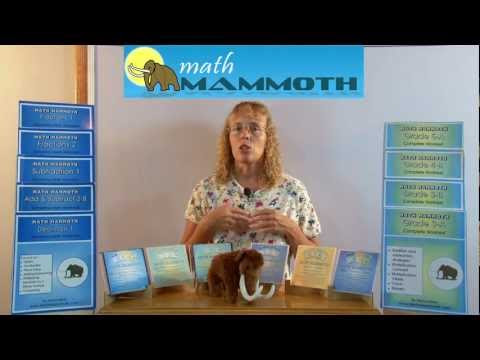## Posts

Showing posts from December, 2011

### Math Mammoth books and their origin - videoHere's a little 2-minute video of me talking about Math Mammoth books, their origin, and also featuring "Mathy" the mammoth. : )

### Fraction videos, part 2

I have created a set of 11 fraction videos that cover simplifying fractions, multiplying fractions by whole numbers, multiplying fractions by fractions, multiplying mixed numbers, fraction multiplication and area, simplify before multiplying, dividing fractions, ratios, and converting fractions to decimals. That's a good bunch of fraction arithmetic! You can use these fraction videos to supplement Math Mammoth Fractions 2 book, or Math Mammoth Grade 5-B complete curriculum. Pre-service or working teachers could use these as lesson plans for fraction topics. Students can of course learn as well, because the videos are meant for both students and teachers. The other "half" of fraction arithmetic is on this page --9 videos. These two pages have a total of 20 videos -  over 3 hours of fraction instruction, for free. It would fill a DVD... but I've uploaded them to Youtube for everyone to enjoy and benefit from.

### Area & perimeter activities - a teacher's experience with Math MammothMs. Pineda used two lessons from my book Mamut Matemáticas Geometría inicial with her bilingual class. Below is her lesson plan, and some reflections. I asked her some questions. How did the children like the activities? The worksheets were a great guide for them because they kept them focused, and the amount of , "What do I do?" questions were very minimal. They seemed comfortable referring back to the worksheets for definitions and examples. I also noticed there was a lot less hand-raising. How did you like using Math Mammoth materials? It definitely made my job easier, because I did not have to continue going back to each group to explain what are or perimeter were. The definition was in front of them along with examples. How many class periods did you use them? It took us two 45-minute periods to complete all the activities. The students were so engaged they did not want to quit. Did you use both English and Spanish, or only Spanish worksheets? I only